Download Presentation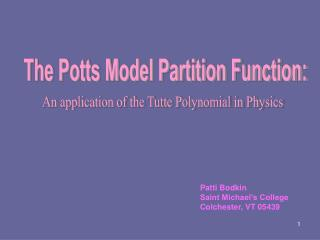The Potts Model Partition Function:

# The Potts Model Partition Function: - PowerPoint PPT Presentation

The Potts Model Partition Function:. An application of the Tutte Polynomial in Physics. Patti Bodkin Saint Michael’s College Colchester, VT 05439. Phase Transitions. Different States of a Model.I am the owner, or an agent authorized to act on behalf of the owner, of the copyrighted work described.
Download Presentation## The Potts Model Partition Function:

An Image/Link below is provided (as is) to download presentation

Download Policy: Content on the Website is provided to you AS IS for your information and personal use and may not be sold / licensed / shared on other websites without getting consent from its author.While downloading, if for some reason you are not able to download a presentation, the publisher may have deleted the file from their server.

- - - - - - - - - - - - - - - - - - - - - - - - - - E N D - - - - - - - - - - - - - - - - - - - - - - - - - -
Presentation Transcript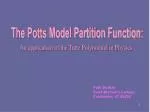The Potts Model Partition Function:

An application of the Tutte Polynomial in Physics

Patti Bodkin

Saint Michael’s College

Colchester, VT 05439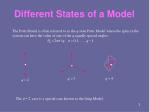Different States of a Model

The Potts Model is often referred to as the q-statePotts Model where the spins in the system can have the value of one of the q equally spaced angles:

The case is a special case known as the Ising Model.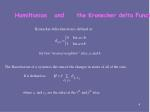Kronecker delta-function is defined as:

for two “nearest neighbor” sites, a and b.

The Hamiltonian of a system is the sum of the changes in states of all of the sites.

It is defined as:

Hamiltonian and the Kronecker delta Function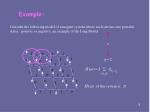of this system is:

Example:

Consider the following model of a magnet system where each site has two possible states, positive or negative, an example of the Ising Model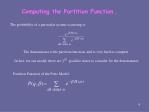In fact, for our model, there are possible states to consider for the denominator.

Computing the Partition Function…

The probability of a particular system occurring is:

Partition Function of the Potts Model: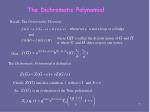whenever is not a loop or a bridge

where is either the disjoint union of and

or where and share at most one vertex

The Dichromatic Polynomial is defined as

then,

Clearly satisfies condition 1, with and .

So, is an evaluation of the Tutte polynomial:

The Dichromatic Polynomial

Recall: The Universality Theorem: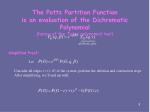Simplified Proof:

Let

Consider all edges of the system, perform the deletion and contraction steps.

After simplifying, we’ll end up with:

The Potts Partition Function

is an evaluation of the Dichromatic Polynomial

(hence of the Tutte polynomial too!)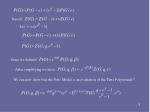Recall:

Let:

Since we defined

After simplifying we have:

We can now show that the Potts Model is an evaluation of the Tutte Polynomial!!Where would you find the Potts Model being used today?

● Atoms

● Animals

● Protein Folds

● Biological Membranes

● Social Behavior

● Phase separation in binary alloys

● Spin glasses

● Neural Networks

● Flocking birds

● Beating heart cellsThe Ising Model and Magnets

At a low temperature, a sheet of metal is magnetized

At high temperatures, the metal becomes less magnetized.

The magnetism of a sheet of metal as it goes through temperature phase transitions can be modeled with the Ising model (Potts Model with q =2 )Magnet Model Phase Transition

Cold Temperature

Hot Temperature

Images taken from applet on:

http://bartok.ucsc.edu/peter/java/ising/keep/ising.htmlNeural Networks

There are two ways to develop machines which exhibit “intelligent behavior”;

Artificial Intelligence Neural Networks

Neural Networks:

Architecture that is based loosely on an animal’s brain.

Learns from a training environment, rather than being preprogrammed.

John Hopfield showed that a highly interconnected network of threshold logic units could be arranged by considering the network to be a physical dynamic system possessing an “energy.”

“Associative Recall” is where a net is started in some initial random state and goes on to some stable final state.

The process of Associate Recall parallel the action of the system falling into a state of minimal energy. The mathematics of these systems is very similar to the Ising Model of magnetic phenomena in materials.Applications of large Q-Potts Model

The extended large Q-Potts Model “captures effectively the global features of tissue rearrangement experiments including cell sorting and tissue engulfment.

The large Q-Potts Model “simulates the coarsening of foams especially in one-phase systems and can be easily extended to include drainage.Resources

Modern Graph Theory, Béla Bollobás

“The Potts Model”, F. Y. Wu

“Chromatic Polynomial, Potts Model and All That”, Alan D. Sokal

Jo Ellis-Monaghan